# Round a Number in Microsoft Excel

In this article we will learn rounding numbers in Microsoft Excel. In Excel we have MROUND, ROUNDUP, ROUNDDOWN, ROUND, INT, CEILING, FLOOR, FIXED, EVEN and ODD etc. which we can use according to the situation and requirement.

If you round a number, the report will not be accurate. If you want to avoid it, show less decimal places without changing the number itself.

First of all we have to understand all formulas individually.

MROUND: - Returns a number rounded to the chosen multiple.

Syntax of “MROUND” function: =MROUND (number, multiple)

Example:Cell A1 contains the number 25

=MROUND (A1, 0.75), function will return 24.75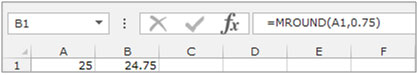ROUNDUP: - This function is used to round up the numbers away from zero.

Syntax of “ROUNDUP” function: =ROUNDUP (number, num_digits)

Example:Cell A1 contains the number 456.25

=ROUNDUP (A1, 0), function will return 457ROUNDDOWN: - This function is used to round down the numbers towards zero.

Syntax of “ROUNDDOWN” function: =ROUNDDOWN (number, num_digits)

Example:Cell A1 contains the number 456.25

=ROUNDDOWN(A1, 0), function will return 456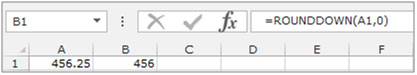ROUND: - This function is used toround a number to a specified number of digits.

Syntax of “ROUND” function: =ROUND (number, num_digits)

Example:Cell A1 contains the number 456.25

=ROUND (A1, 0), function will return 456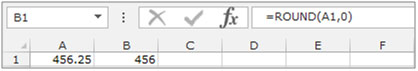INT: - This function is used round a number down to the nearest integer.

Syntax of “INT” function: =INT (number)

Example:Cell A1 contains the number 456.25

=INT (A1), function will return 456CEILING: - This function is used to round a number up to the nearest multiple of significance.

Syntax of “CEILING” function: =CEILING (number, significance)

Example:Cell A1 contains the number 456.25

=CEILING (A1, 0.75), function will return 456.75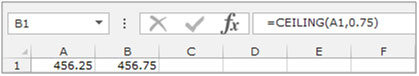FLOOR: - This function is used to round a number down to the nearest multiple of significance.

Syntax of “FLOOR” function: =FLOOR (number, significance)

Example:Cell A2 contains the number 456.25

=FLOOR (A2, 0.75), function will return 456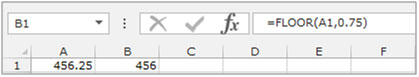FIXED: - This function is used round a number to the specified number of decimals and returns the result as text with or without commas.

Syntax of “FIXED” function: =FIXED (number,[decimals],[no_commas])

Example:Cell A1 contains the number 456.25

=FIXED (A1,1,TRUE), function will return 456.3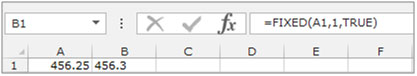EVEN: - This function is used to round a positive number up and negative number down to the nearest even integer.

Syntax of “EVEN” function: =EVEN (number)

Example:Cell A1 contains the number 456.25

=EVEN (A1), function will return 458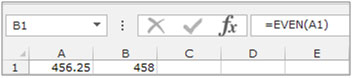ODD: - This function is used to round a positive number up and negative number down to the nearest odd integer.

Syntax of “ODD” function: =ODD (number)

Example:Cell A2 contains the number 456.25

=ODD(A1), function will return 457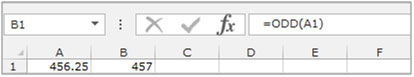Let’s take an example and understand how we can round a number up and down to the nearest 100.

I have 2 numbers 1445 and 1175. I want 1445 to be rounded down to 1400 and 1175 should become 1200. The numbers are already integers.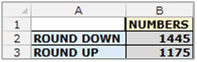There are 3 ways in excel to resolve this problem. You can solve usingFLOOR, MROUNDand ROUND functions.

Let’s resolve first with the “FLOOR” function:-

• Select the cell C2 and write the formula.
• =FLOOR (B2+49,100) press enter on the keyboard.
• Copy the same formula and paste into cell C3.
• The function will return the rounded figure as per the requirement.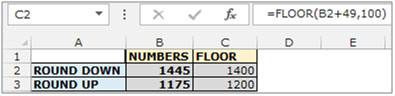“MROUND” function:-

• Select the cell D2 and write the formula.
• =MROUND (B2, 100) press enter on the keyboard.
• Copy the same formula and paste into cell D3.
• The function will return the rounded figure as per the requirement.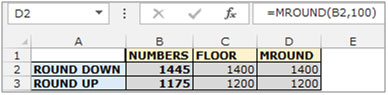“ROUND” function:-

• Select the cell E2 and write the formula.
• =ROUND (B2,-2)press enter on the keyboard.
• Copy the same formula and paste into cell E3.
• The function will return the rounded figure as per the requirement.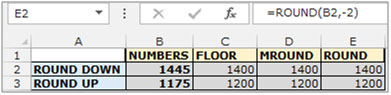Theseare the ways you can round up and round down the numbers till 2 digits or more as per the requirement.

## Users are saying about us...

1.Can you tell me how to round this:

Example:

if result in one cell show 1550 roundup to 1600 or in the same cell if result show 1540 rounddown to 1500

I am glade to here from you soon.

Thanks Exceltip.com

2.Hi Sophannasoth,

Assume you have numbers in cell A1 and A2, use the below function in cell B1 and paste the same in cell B2 you will get required result by using this formula...
=Floor(A1+49,100)

Regards
Excel Tip

3.Thirpal Das Khatri

Very useful for beginners

Terms and Conditions of use

The applications/code on this site are distributed as is and without warranties or liability. In no event shall the owner of the copyrights, or the authors of the applications/code be liable for any loss of profit, any problems or any damage resulting from the use or evaluation of the applications/code.